Fluids Index

Drag on objects moving through fluids

Introduction..... Symbols..... Boundary layer..... Drag..... Drag 2D objects..... Drag - 3D objects .....

Introduction
Fluid Flow

Fluid flowing past an object tends to drag the object along in the direction of fluid flow.  If an object is moving through a stationary fluid the drag tends to slow the object down.  If the object is stationary in a flowing fluid the drag tends to move the object in the direction of flow.  The drag comprises two components:

- Pressure drag or form drag which is based on the pressure difference between the upstream and downstream surfaces of the object

- Skin Friction which results from the viscous shear of the fluid flowing over the object surfaces.

The form drag is the resultant of resolved forces normal to the surface of the object and the skin-friction is the resultant of resolved forces tangential to the surface.   The total drag on an object is called the profile drag and is the sum of the pressure and skin-friction drag
When the drag is primarily viscous drag, the body is streamlined, and when the drag force is primarily pressure drag the body is called a bluff body.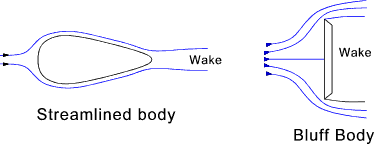A perfect fluid flowing past an infinitely long cylinder is represented as streamlines which are arranged such that the flow through each streamline is fixed at Q .  The streamlines flow over the cylinder and all forces are balanced front/back and top /bottom and there is therefore no form drag.  A perfect fluid cannot transfer shear stress so there is no viscous drag.  In real fluids there is a pressure build up on the front surface as the fluid is slowed and the streamlines are re directed round the cylinder.   As the fluid flows over the cylinder the fluid separates into a wake which is a lower pressure region. There is therefore significant form drag.   There is also skin-friction drag as the fluid passes over the surface.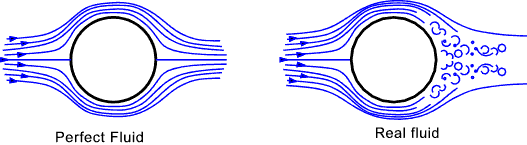If the cylinder rotates, as shown below, the drag between the surface and the fluid results in the fluid flow as shown.  The flow results in higher fluid velocities above the cylinder compared with the flow below the cylinder .  Application of bernoulli's equation results a lower pressure above the cylinder and a consequent lift.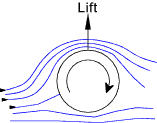Symbols
 a = Acceleration (m/s2 A = Area (m2) a = Speed of sound (m/s) C D =Drag coefficient F = Force (N) g = acceleration due to gravity (m/s2 ) h = fluid head (m) K = Bulk modulus (MPa ) m = mass (kg) M = mach number u /a M = Molecular weight p = fluid pressure (N /m2 ) p abs - absolute pressure (N /m2 ) p gauge - gauge pressure (N /m2 ) p atm - atmospheric pressure (N /m2 ) p s= surface pressure (N /m2 ) Q = Volume flow rate (m3 /s) Re = Reynolds Number = u.ρD/μ Re x = Local Reynolds Number = u.ρD/μ ρ = fluid density (kg /m2 ) s = specific volume (m3 /kg) u = fluid velocity (m/s) u n = Normal flow fluid velocity (m/s) v = fluid velocity (m/s) θ =slope (radians) ρ = density (kg/m3) ρ r = density (kg/m3) τ = shear stress (N /m2) μ = viscosity (Pa.s) ν kinematic viscosity (m2�s-1) υ = Specific volume (m3 / kg)

Boundary Layer and Wake

Flow past a slender body or flat plate arranged parallel to the flow results in a flow regime as shown below.  The flows at the surface are brought to rest relative to the surface and flows close to the surface are slowed.   This effect reduces rapidly as the distance from the surface increases until the flow is completely unaffected.  The flow pattern may be split into two regions: A thin boundary layer in which friction (viscous) drag forces are important and a region in which bernoulli's equation primarily applies i.e. the total head is constant.

The boundary layer concept where the influence of viscosity is concentrated bridges the gap between classical hydrodynamics based on inviscid fluids and bernoulli's theorem and the behaviour of real fluids.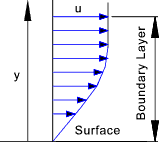The boundary layer as formed by a fluid flowing along one side of the flat plate is initially laminar, as shown below. The velocity gradients are primarily due to the viscous drag near the surface of the plate.  The velocity upstream of the plate and in the region outside the boundary layer is u n .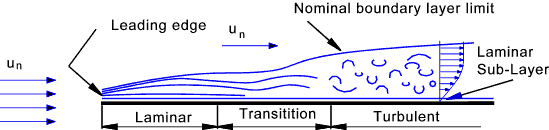At the leading edge of the plate the fluid is retarded and the boundary layer is initiated.  As more fluid is slowed because of the viscous forces the boundary layer thickens.  Initially the boundary layer includes only laminar flow but as the boundary layer thickness increases the laminar layer loses stability and the flow becomes less even.  The point at which the laminar flow starts to deteriorate is called the transition point and is at the start of a region called the transition region where the flow changes from laminar flow to turbulent flow.   At the plate surface a thin laminar sub-layer remains below the turbulent boundary layer.  The sketch shows the boundary layer thickness very much magnified relative to the length along the plate.  The thickness of the boundary layer on an aircraft wing may only be a few mm thick.

Consider a fluid flow over a curved surface as shown below.  The pressure of the fluid is initially higher at the approach (A) compared to that when the fluid has accelerated over the surface (B).   This is because the pressure head falls as the velocity head rises.   When the fluid starts to decelerate as is moves over the surface the pressure rises from B to C to D.   The initial pressure change from A->B assists the flow over the surface and the pressure gradient is favourable.  However the pressure gradient from B- > C -> D is not favourable and there is a tendency for the pressure to retard the boundary layer.  The effect of this is greater at the solid -fluid surface because the local momentum of the fluid is least.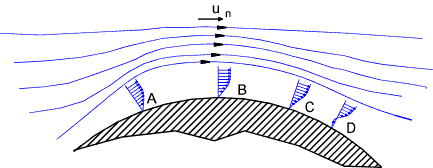There is a point where the reverse pressure causes sufficient flow that the boundary layer velocity gradient becomes zero and there is a tendency for the fluid to separate.  In the figure above this is shown at point C and is called the point of laminar separation.   The result of this, so called,separation is to impair the process of conversion between kinetic energy and pressure energy with internal fricitonal losses to heat.   A laminar boundary layer has less kinetic energy compared to a turbulent boundary layer and this effect is reduced if a turbulent boundary layer flow can be encouraged.  A golf ball is covered with dimples to encourage a turbulent boundary layer and therefore delay the onset of separation

The region of constant reduced pressure behind the at the rear of an object, called the wake, lies beyond the separation point and is responsible for the pressure drag.

A streamline shape is such a form that the change in velocity head downstream of the maximum velocity is reduced such that the separation point is moved as far back as possible .see figure below.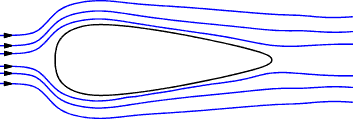Drag notes.

When calculating the total drag a very simple equation is used as shown below.  This equation is not valid for relative fluid velocities approaching sonic velocity.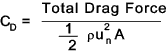For bluff bodies A = The frontal area of the body facing the flow.  For thin flat plates and similar shaped items subject to primarily skin-friction A = the total surface area swept by the fluid flow (both sides)   For wings in aeronautical calculations A is the product of the wing span and the mean wing chord.

The denominator is simply the product of the dynamic head of the undisturbed fluid [(1/2) ρ u n2 ] and the specified area.

The flow patterns in the wake are dependent on the Reynolds number as illustrated by considering an infinitely long cylinder in a fluid flow providing a two dimensional flow pattern.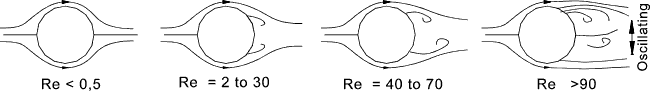At low reynolds numbers (Re < 0,5)the inertial forces are negligible compared to the viscous forces and the streamlines come together behind the cylinder.   The drag is therefore roughly proportional to u n. Drag is inversely proportional to C D.

At reynolds number 2 to 30 a wake is formed behind the cylinder but the streamlines come together behind the cylinder.  Eddies form which rotate in opposite direction

At Reynolds numbers 40 to 70 the eddies elongates and wake flow instability inititiates.  At high Reynolds numbers eddies form and break off each side of the cylinder alternately.

At higher levels (Re > 90 ) the eddies form vortices downstream of the cylinder.  This unsymetrical flow pattern causes telephone wires to sing in the wind, cause venetian blinds to flutter and are a major design problem for tall chimneys. This arrangement of vortices is known as a Karman vortex street.

The drag drag coefficient C D reaches a minimum of about 0,9 at Re = 2000 and then increases a little because the turbulance of the wake increases and the position of separation gradually moves upstream.   At this stage the profile drag is nearly all due to pressure (form) drag.

At an Re value of about 20 x 105 the laminar boundary layer becomes turbulant before separation.   The turbulant boundary layer has higher kinetic energy than the laminar based layer and is better able to withstand the adverse pressure gradiant.   There is therefore a sudden drop in the drag down to about 0,3.   As the Reynold number rises there is a conequent increase in the drag coefficient to about 0,7.   For increases in Reynold number above 4 x 106 the drag is independent of the Re number.

Drag for Infinitely long PLATE and infinitely long CYLINDER

Drag calculated is better calculated using the variation of the drag equation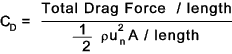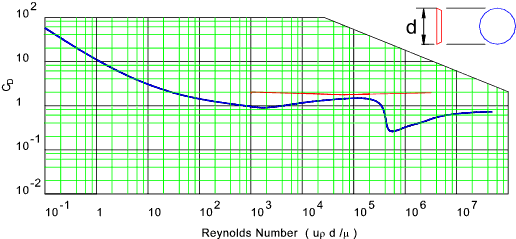The drag coefficient for a shape which is finitely long is affected by fluid flow around the ends.  This is illustrated by considering the plate for reynolds number above 1000. The drag coefficient varies as follows for different Length(L) to Breadth (d) ratios.

 L /d 1 2 4 10 18 Infinite C D 1,1 1,15 1,19 1,29 1,4 2,01

Drag for long plate parallel to flow and streamlined strut

The drag coefficient is mainly friction drag (C f ).    The area is the length x width (l.d )
Drag calculated is better calculated using the variation of the drag equation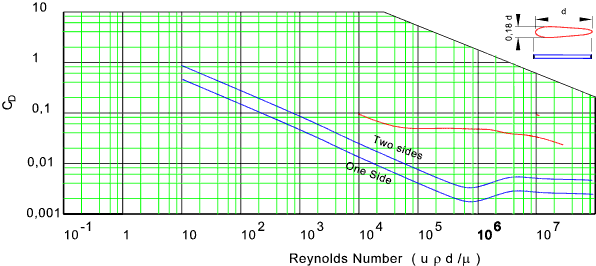Drag factor for various long objects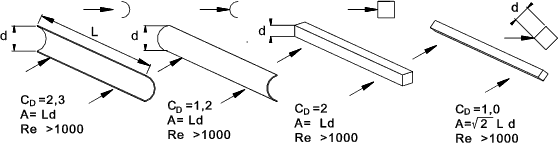Drag for DISC and SPHERE<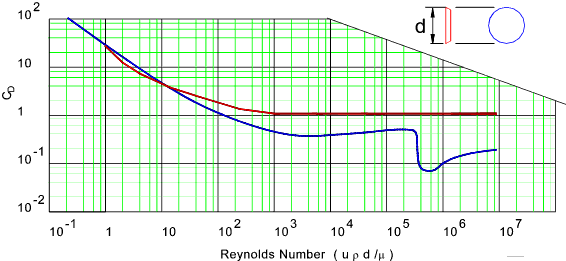Drag factor for various objects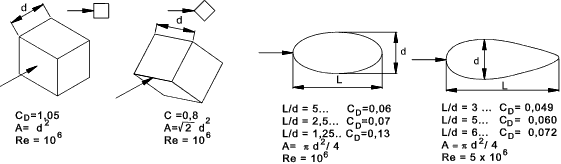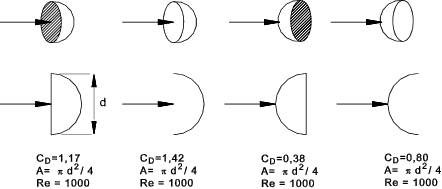Drag for a human body = C D = 1 to 1,3

Drag for a normal car = C D = 0,37

 Useful Links Drag of Blunt Bodies and Streamlined Bodies.. Concise and clear set of notes - Very easy to understand Encyclopedia article -Fluid Mechanics Drag.. Very informative article.. Drag force and drag ceofficients.. Download of lecture notss.. Engineers toolbox drag coefficients.. Notes and a table.. Wikipedia drag coefficients.. Very detailed nots and tables..

Fluids Index